# ILP Part 20 — Students Placement Problem Part 3 — Useful constraints

This is the twentieth part of the ILP series. For your convenience you can find other parts in the table of contents in Part 1 – Boolean algebra

Last time we defined necessary constraints for students placement problem. Today we are going to implement something similar. We will still focus on defining requirements for schedule and ignoring the cost function, however, this time we will implement constraints not required for finding feasible solution yet very useful. Apart from the constraints, which are necessary for finding a schedule allowing the students to complete their studies, we can imagine many constraints, whose goal is to improve the quality of the schedule. Let us begin.

# Lower Bound for Assigned People

Just like we added constraint for maximum number of students assigned to a single class, we can do the same for minimum number. This feature is very helpful for lecturers because it allows to create groups with almost the same number of students. In order to achieve this, we can use the following constraint: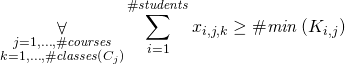# Class Sizes Divisible by Team Sizes

From time to time lecturer wants to divide students into teams of equal size, for instance to let them work together on a single project. If we want to add this requirement, we can use the following formula: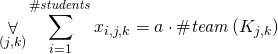for each class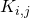where students should create teams (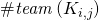means the size of a team).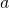is any integer.

# Removing classes

So far, we assumed that we use all classes from the schedule provided by the authorities of university. However, we might imagine a different situation — for instance we might want to remove some classes. Let us say that we have 60 places for one course but only 30 registered people. We might want to remove some classes in order to have bigger groups and utilize lecturer’s time better. Of course we could just arbitrarily select classes to remove but it would be better if the solver would remove the least wanted ones.

In other words, we want to add constraints which will force the solver to remove the most unwanted classes from the solution. But we also need to remember that we added constraints for having minimum number of assigned students so we need to be careful.

First, we need to add a boolean variable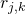meaning that class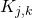is removed. Let us assume that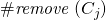denotes the total number of classes in course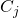which need to be removed (of course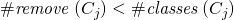. The constraint to remove classes is: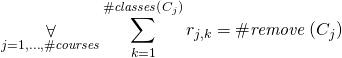This formula says that the sum of variables representing classes’ removal must be equal to the total number of classes which should be removed. Now we need to change our constraint for lower bound on the number of people assigned to a class to the following: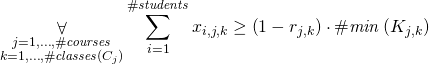We can do this, because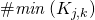is a constant value, so we can multiply it easily by variable.

So how does it work? Whenis(which means that classis removed), lower bound on the number of people count assigned to that class cannot be less than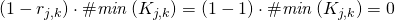, so it can by any non-negative integer. On the other hand, whenis equal to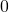, it works just like before.

Now we can remove a class and not bother with its participation lower bound. But we still can assign students to removed classes. We need to change our formula for room capacity, but we can do it in the same way: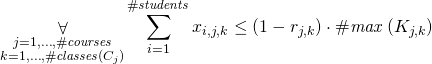# Summary

Most of the defined requirements are rather straightforward and yet very powerful. If we would solve the problem right now, the solution would represent very handy assignment, however, students still would not be satisfied. Next time we are going to implement basic cost function to improve their happiness.